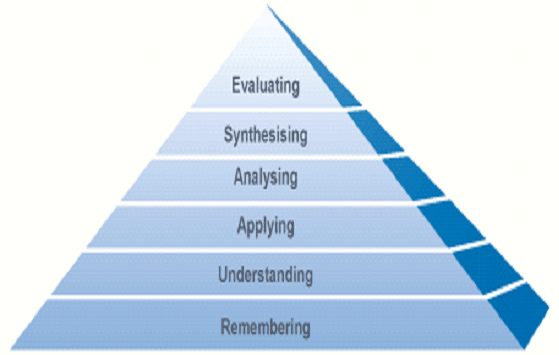# Generating Curiosity in Mathematics Learning

Published February 2011.

Music teachers challenge students to listen and participate.
English and History teachers invite students to journey in other worlds.
Art and Drama teachers offer students opportunities to explore.
What are we to offer students if they are to function mathematically?

What do you understand by 'functioning mathematically'?
What characteristic behaviours do your highly achieving mathematicians exhibit?

## Three key characteristics

• working systematically
• generalising
• proving and explaining
 Many students Highly attaining students work randomly work systematically notice obvious patterns $\quad \quad$ conjecture and generalise make statements offer explanations and proofs

### What do these behaviours look like in practice?

Many numbers can be expressed as the sum of two or more consecutive integers.

$15 = 7 + 8$
$15 = 4 + 5 + 6$
$15 = 1 + 2 + 3 + 4 + 5$

Look at numbers other than 15 and find out all you can about writing them as sums of consecutive whole numbers.

Working randomly:

$5=2+3$
$4+5+6+7=22$
$1+2+3+4+5+6=21$
$11=5+6$

Working systematically:

 $2$ $3$ $4$ $1=0+1$ $\quad \quad$ $2=$ $3=1+2$ $0+1+2$ $\quad \quad$ $4=$ $5=2+3$ $6=$ $1+2+3$ $0+1+2+3$ $7=3+4$ $8=$ $9=4+5$ $2+3+4$ $10=$ $1+2+3+4$

Noticing obvious patterns
• Odd numbers can be written as two consecutive numbers.
• Multiples of $3$ can be written as three consecutive numbers.
• Even numbers can be written as four consecutive numbers.
Conjecturing and generalising
• Multiples of $3$ can be written as the sum of three consecutive numbers.
• Multiples of $5$ can be written as the sum of five consecutive numbers.
• I wonder if multiples of $7$ can be written as the sum of seven consecutive numbers'?¦
• I wonder if multiples of $9$ can be written as the sum of nine consecutive numbers'?¦
• I wonder if multiples of $x$ (where $x$ is odd), can be written as $x$ consecutive numbers'?¦
Making statements
• Multiples of $3$ can be written as three consecutive numbers.
• If you give me any multiple of three, I can tell you the three numbers by dividing by three and that will be the middle number.
• Even numbers can be written as four consecutive numbers.
Offering explanations and proofs
• If you give me three consecutive numbers I can always turn them into a multiple of three.
• $x + (x+1) + (x+2) = (x+1) + (x+1) + (x+1) = 3 (x+1)$
• Same will apply to five, seven, nine and any odd number because you can pair off numbers on either side of the middle number.

## Creating opportunities for students to develop their mathematical thinking

• Promoting a conjecturing atmosphere
• Careful use of questions and prompts
• Low threshold high ceiling tasks
• Modelling behaviour
• Whole class discussion
• Highlighting behaviour that you want to promote
• HOTS not MOTS

### Promoting a conjecturing atmosphere

• Accepting 'messy' work
• Valuing risk-taking and half-formed ideas
• Encouraging discussion
• Providing thinking time

### Careful use of questions and prompts

• What have you found out so far?
• Do you notice anything?
• Is that always true?
• Can you convince us?
• Can anyone think of a counter example?
• What if...?
• What might you try next?
• Is there a way you could organise your findings?

### Low threshold high ceiling tasks

• Using problems that everyone can 'get into' but which also offer opportunities for working at a higher level
• There is no need to 'label' students in advance

### Modelling behaviour

Students should be in the presence of a teacher who is
• curious
• intrigued
• wanting to find out more
• appreciates the value of proof
• expects to understand why things happen

### Whole class discussion

• Early on: to share
• "opening gambits"
• Half way through: to share
• possible findings
• useful strategies
• new questions
• Towards the end: to share
• conclusions
• explanations
• proofs

### Highlighting behaviour that you want to promote

Drawing attention to and valuing process as well as outcome.

### HOTS not MOTS

H igher O rder T hinking S kills

not

M ore o f t he S ameEach task requiring lower order thinking has a higher order thinking equivalent/partner. Identify which is which and sort them into pairs. (see Lower and Higher Order Thinking document)

#### How can you generate questions that promote these HOTS in a mathematical context?

• Ask students to work backwards
• Set questions which have a variety of solutions
• Set questions which can be solved in a variety of ways

#### Ask students to work backwards

Find the area and perimeter of a $3cm \times 8cm$ rectangle
If the area of a rectangle is $24$ cm² and the perimeter is $22$ cm, what are its dimensions?

Find the area and perimeter of a $3cm \times 8cm$ rectangle
What if the area of a rectangle (in cm²) is equal to the perimeter (in cm), what could its dimensions be?

#### Set questions which have a variety of solutions

Find the perimeter of a $3cm \times 8cm$ rectangle
Find a rectangle which has unit sides and a perimeter of $100$ .
How many answers are there and how do you know you've got them all?

Set questions which can be solved in a variety of ways
Find the area and perimeter of a $3cm \times 8cm$ rectangle.
If the area of a rectangle is $24$cm² and the perimeter is $22$cm, what are its dimensions? How did you work this out?